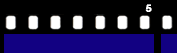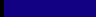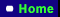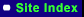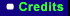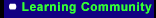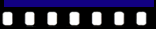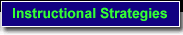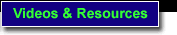NCTM Standards & Expectations

Find MathVIDS Teaching Plans that address the following NCTM Standards & Expectations:

 Number and Operations Algebra Standard: Understand numbers, ways of representing numbers, relationships among numbers, and number systems Standard: Understand patterns, relations, and functions Standard: Understand meanings of operations and how they relate to one another Standard: Represent and analyze mathematical situations and structures using algebraic symbols Standard: Compute fluently and make reasonable estimates

 Number and Operations Standard: Understand numbers, ways of representing numbers, relationships among numbers, and number systems
 Pre-K–2 Expectations: In prekindergarten through grade 2 all students should– • count with understanding and recognize "how many" in sets of objects; Related MathVIDS Teaching Plans: • use multiple models to develop initial understandings of place value and the base-ten number system; Related MathVIDS Teaching Plans: • develop understanding of the relative position and magnitude of whole numbers and of ordinal and cardinal numbers and their connections; Related MathVIDS Teaching Plans: • develop a sense of whole numbers and represent and use them in flexible ways, including relating, composing, and decomposing numbers; Related MathVIDS Teaching Plans: Grades 3–5 Expectations: In grades 3–5 all students should– • understand the place-value structure of the base-ten number system and be able to represent and compare whole numbers and decimals; Related MathVIDS Teaching Plans: • recognize equivalent representations for the same number and generate them by decomposing and composing numbers; Related MathVIDS Teaching Plans: • use models, benchmarks, and equivalent forms to judge the size of fractions; Related MathVIDS Teaching Plans: • recognize and generate equivalent forms of commonly used fractions, decimals, and percents; Related MathVIDS Teaching Plans:

Standard: Understand meanings of operations and how they relate to one another

 Grades 3–5 Expectations: In grades 3–5 all students should– • understand the effects of multiplying and dividing whole numbers; Related MathVIDS Teaching Plans:

Standard: Compute fluently and make reasonable estimates

 Pre-K–2 Expectations: In prekindergarten through grade 2 all students should– • develop and use strategies for whole-number computations, with a focus on addition and subtraction; Related MathVIDS Teaching Plans: • develop fluency with basic number combinations for addition and subtraction; Related MathVIDS Teaching Plans: Grades 3–5 Expectations: In grades 3–5 all students should– • develop fluency with basic number combinations for multiplication and division and use these combinations to mentally compute related problems, such as 30x50; Related MathVIDS Teaching Plans: • develop fluency in adding, subtracting, multiplying, and dividing whole numbers; Related MathVIDS Teaching Plans: • develop and use strategies to estimate the results of whole-number computations and to judge the reasonableness of such results; Related MathVIDS Teaching Plans: • use visual models, benchmarks, and equivalent forms to add and subtract commonly used fractions and decimals; Related MathVIDS Teaching Plans:
 Algebra Standard: Understand patterns, relations, and functions
 Pre-K–2 Expectations: In prekindergarten through grade 2 all students should– • sort, classify, and order objects by size, number, and other properties; Related MathVIDS Teaching Plans: • recognize, describe, and extend patterns such as sequences of sounds and shapes or simple numeric patterns and translate from one representation to another; Related MathVIDS Teaching Plans:

Standard: Represent and analyze mathematical situations and structures using algebraic symbols

 Grades 3–5 Expectations: In grades 3–5 all students should– • identify such properties as commutativity, associativity, and distributivity and use them to compute with whole numbers; Related MathVIDS Teaching Plans: